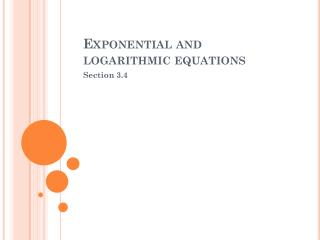DownloadDownload PresentationExponential and logarithmic equations

# Exponential and logarithmic equations

Télécharger la présentation## Exponential and logarithmic equations

- - - - - - - - - - - - - - - - - - - - - - - - - - - E N D - - - - - - - - - - - - - - - - - - - - - - - - - - -
##### Presentation Transcript

1. Exponential and logarithmic equations Section 3.4

2. Exponential & Log Equations • In the previous sections, we covered: • Definitions of logs and exponential functions • Graphs of logs and exponential functions • Properties of logs and exponential functions • In this section, , we are going to study procedures for solving equations involving logs and exponential equations

3. Exponential & Log Equations • In the last section, we covered two basic properties, which will be key in solving exponential and log equations. • One-to-One Properties • Inverse Properties

4. Exponential & Log Equations • We can use these properties to solve simple equations:

5. Exponential & Log Equations • When solving exponential equations, there are two general keys to getting the right answer: • Isolate the exponential expression • Use the 2nd one-to-one property

6. Exponential & Log Equations • Solve the following equation: Isolate the exponential expression: Apply the 2nd one-to-one property

7. Exponential & Log Equations • Solve the following equation: Isolate the exponential expression: Apply the 2nd one-to-one property

8. Exponential & Log Equations • Solve the following equation: Isolate the exponential expression: Apply the 2nd one-to-one property

9. Exponential & Log Equations • Solve the following equations:

10. Exponential & Log Equations • Solving Equations of the Quadratic Type • Two or more exponential expressions • Similar procedure to what we have been doing • Algebra is more complicated

11. Exponential & Log Equations • Solve the following equation: Start by rewriting the equation in quadratic form. Factor the quadratic equation:

12. Exponential & Log Equations

13. Exponential & Log Equations • Solve the following equation:

14. Exponential and logarithmic equations Section 3.4

15. Exponential & Log Equations • Solve the following equation:

16. Exponential & Log Equations • Solve the following equation: Since these are exponential functions of a different base, start by taking the log of both sides

17. Exponential & Log Equations

18. Exponential & Log Equations • So far, we have solved only exponential equations • Today, we are going to study solving logarithmic equations • Similar to solving exponential equations

19. Exponential & Log Equations • Just as with exponential equations, there are two basic ways to solve logarithmic equations • Isolate the logarithmic expression and then write the equation in equivalent exponential form • Get a single logarithmic expression with the same base on each side of the equation; then use the one-to-one property

20. Exponential & Log Equations • Solve the following equation: Isolate the log expression: Rewrite the expression in its equivalent exponential form

21. Exponential & Log Equations • Solve the following equation. Get a single log expression with the same base on each side of the equation, then use the one-to-one property

22. Exponential & Log Equations • Solve the following equation Isolate the log expression: Rewrite the expression in exponential form

23. Exponential & Log Equations • In some problems, the answer you get may not be defined. • Remember, is only defined for x > 0 • Therefore, if you get an answer that would give you a negative “x”, the answer is considered an extraneous solution

24. Exponential & Log Equations • Solve the following equation Isolate the log expression: Rewrite the expression in exponential form

25. Exponential & Log Equations Would either of these give us an undefined logarithm?

26. Exponential & Log Equations • Solve the following equations:

27. Exponential and logarithmic equations Section 3.4 - Applications

28. Exponential & Log Equations • Solve the following equation:

29. Exponential & Log Equations • Solve the following equation:

30. Exponential & Log Equations

31. Exponential & Log Equations • How long would it take for an investment to double if the interest were compounded continuously at 8%? What is the formula for continuously compounding interest? If you want the investment to double, what would A be?

32. Exponential & Log Equations It will take about 8.66 years to double.

33. Exponential & Log Equations • You have deposited \$500 in an account that pays 6.75% interest, compounded continuously. How long will it take your money to double?

34. Exponential & Log Equations • You have \$50,000 to invest. You need to have \$350,000 to retire in thirty years. At what continuously compounded interest rate would you need to invest to reach your goal?

35. Exponential & Log Equations • For selected years from 1980 to 2000, the average salary for secondary teachers y (in thousands of dollars) for the year t can be modeled by the equation: y = -38.8 + 23.7 ln t Where t = 10 represents 1980. During which year did the average salary for teachers reach 2.5 times its 1980 level of \$16.5 thousand?

36. Exponential & Log Equations

37. Exponential & Log Equations

38. Exponential & Log Equations